Important Questions: Exponents and Powers

# Important Questions: Exponents and Powers

Test Description

## 20 Questions MCQ Test Mathematics (Maths) Class 7 | Important Questions: Exponents and Powers

Important Questions: Exponents and Powers for Class 7 2022 is part of Mathematics (Maths) Class 7 preparation. The Important Questions: Exponents and Powers questions and answers have been prepared according to the Class 7 exam syllabus.The Important Questions: Exponents and Powers MCQs are made for Class 7 2022 Exam. Find important definitions, questions, notes, meanings, examples, exercises, MCQs and online tests for Important Questions: Exponents and Powers below.
Solutions of Important Questions: Exponents and Powers questions in English are available as part of our Mathematics (Maths) Class 7 for Class 7 & Important Questions: Exponents and Powers solutions in Hindi for Mathematics (Maths) Class 7 course. Download more important topics, notes, lectures and mock test series for Class 7 Exam by signing up for free. Attempt Important Questions: Exponents and Powers | 20 questions in 20 minutes | Mock test for Class 7 preparation | Free important questions MCQ to study Mathematics (Maths) Class 7 for Class 7 Exam | Download free PDF with solutions
 1 Crore+ students have signed up on EduRev. Have you?
Important Questions: Exponents and Powers - Question 1

### 23+23+23+23 is equal to ____.

Detailed Solution for Important Questions: Exponents and Powers - Question 1

We have
23+23+23+2= 4 × (23
= 2× 2= 25

Important Questions: Exponents and Powers - Question 2

### The value of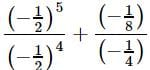is ____.

Detailed Solution for Important Questions: Exponents and Powers - Question 2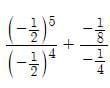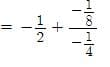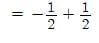= 0

Important Questions: Exponents and Powers - Question 3

### {(1/3)−3−(1/2)−3} ÷ (1/4)−3 ?

Detailed Solution for Important Questions: Exponents and Powers - Question 3

{(1/3)−3−(1/2)−3} ÷ (1/4)−3 = {(3)3−(2)3} ÷ (4)3 = {27−8} ÷ 64 = 19/64

Important Questions: Exponents and Powers - Question 4

The value of (−8) × (−8) × (−8) × (−8) × (−8) + (−8) × (−8) × (−8) × (−8) × (−8) is ____.

Detailed Solution for Important Questions: Exponents and Powers - Question 4

(−8)5+ (−8)= −2×(8)5 = −2×(2)15

= −(2)16 = −(4)8

Important Questions: Exponents and Powers - Question 5

If (5/3)−5 × (5/3)11 = (5/3)8+x, then x = ?

Detailed Solution for Important Questions: Exponents and Powers - Question 5

(5/3)−5 × (5/3)11 = (5/3)8+x
⇒ (5/3)−5+11 = (53)8+x

⇒ (5/3)6 = (5/3)8+
On comparing, we get,
8 + x = 6

⇒ x = −2

Important Questions: Exponents and Powers - Question 6

The value of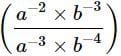is ________.

Detailed Solution for Important Questions: Exponents and Powers - Question 6=(a−2+3 × b−3+4)

= a×b

= ab

Important Questions: Exponents and Powers - Question 7

{(3/4)−1−(1/4)−1}−1 = ?

Detailed Solution for Important Questions: Exponents and Powers - Question 7

{(3/4)−1−(1/4)−1}−1 = {4/3−4}−1 = {−8/3}−1=−3/8

Important Questions: Exponents and Powers - Question 8

Express the given number using exponential notation: 3125

Detailed Solution for Important Questions: Exponents and Powers - Question 8

3125 = 5 × 5 × 5 × 5 × 5 = 55

Important Questions: Exponents and Powers - Question 9

If (5/9)4 × (5/9)−10 = (5/9)−4(5/9)2a−1,then the value of a is _____.

Detailed Solution for Important Questions: Exponents and Powers - Question 9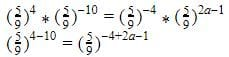Equating the powers,
-6=2a-5
2a=-1
a=-1/2

Important Questions: Exponents and Powers - Question 10

Which of the following is equivalent to 7.7×10−6 ?

Detailed Solution for Important Questions: Exponents and Powers - Question 10

7.7×10−6 = 7.7/100000 = 0.0000077

Important Questions: Exponents and Powers - Question 11

If (3/2)2 × (3/2)a+5 = (3/2)8, then a = ____.

Detailed Solution for Important Questions: Exponents and Powers - Question 11

(3/2)× (3/2)a+5 = (3/2)8
⇒(3/2)a+7 = (3/2)8
On comparing, we get,
a+7 = 8 ⇒ a =1

Important Questions: Exponents and Powers - Question 12

The number which is multiplied by (−8)−1 to obtain a product equal to 10−1 is ____.

Detailed Solution for Important Questions: Exponents and Powers - Question 12

Let the number be x, Then, (−8)−1× x = (10)−1

⇒(−1/8) × x = (1/10) ⇒ x= −4/5

Important Questions: Exponents and Powers - Question 13

The value of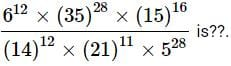Detailed Solution for Important Questions: Exponents and Powers - Question 13

Correct Answer :- b

Explanation :  [(2*3)12 * (5*7)28 * (5*3)16]/[(7*2)12 * (7*3)11 * 528]

= [(2)12 * (3)12 * (5)28 * (7)28 * (5)16 * (3)16]/[(7)12 * (2)12 * (7)11 * (3)11 * (5)28]

= [(2)12 * (3)12+16 * (5)28+16 * 316]/[(7)12+11 * (2)12 * (3)11 * (5)28]

= [(2)12 * (3)28 * (5)44 * 316]/[(7)23 * (2)12 * (3)11 * (5)28]

= 317 * 516 * 75

Important Questions: Exponents and Powers - Question 14

The value of a− ba, if a = 3, b = 7 is

Detailed Solution for Important Questions: Exponents and Powers - Question 14

ab − ba =37−7= 2187−343 = 1844

Important Questions: Exponents and Powers - Question 15

The value of (23/25)× (−1/2)×2× (3/4)2 is

Detailed Solution for Important Questions: Exponents and Powers - Question 15

(23/25)× (−1/2)×2× (3/4)2

=1×(−1)× (2)−5 × 2× 3× (2)−4=−9/64

Important Questions: Exponents and Powers - Question 16

A rectangular piece of land is to be sold off in smaller pieces. The total area of the land is 217 sq. miles. The pieces to the cut out are 162 sq. miles in size. How many smaller pieces of the land can be sold at the given size?

Detailed Solution for Important Questions: Exponents and Powers - Question 16

Required number of pieces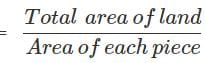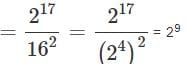Important Questions: Exponents and Powers - Question 17

Pooja spends about Rs.40000 each month on utensils hold. If she has about 1×10utensils, what is the total amount of money (in Rs.) spent on the households in one month?

Detailed Solution for Important Questions: Exponents and Powers - Question 17

Total amount of money spent Rs.(40000×1×108) = Rs.(4.0×1012)

Important Questions: Exponents and Powers - Question 18

All the planets revolve around the Sun in elliptical orbits. Uranus's farthest distance from the Sun is approximately 3.004×109 km and its closest distance is approximately 2.7499×109 kmkm. What is the average distance of Uranus from the Sun?

Detailed Solution for Important Questions: Exponents and Powers - Question 18

Distance of Uranus from Sun =(3.004×109)km
Uranus closest distance from Sun =(2.7499×109)km
Total distance = 3.004×10+ 2.7499×109
= (5.753×109)km
Average distance = (5.753×109)/2
= (2.8765×109)km

Important Questions: Exponents and Powers - Question 19

There are three places A, B, C in a straight line as shown below. If distance between place A and B is (2.4×106)m and distance between 8 and C is (5.2×105)m, then find the distance between place A and C in standard form.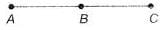Detailed Solution for Important Questions: Exponents and Powers - Question 19

Distance between place A and B
= 2.4×106m = 2400000 m
Distance between place 6 and C = 5.2×105 m = 5.2×100000 m = 520000 m
Distance between place A and C
= (2.4×10+ 5.2×105)m = (2400000 + 520000)m = 292×104m
Standard form of 292×104m = 2.92×106

Important Questions: Exponents and Powers - Question 20

Mr Gupta asked two of his students to solve the expression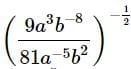and write the answer on blackboard. Aryan wrote the answer as 3a−4bwhereas Ayan wrote the answer as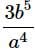. Who wrote the correct answer?

Detailed Solution for Important Questions: Exponents and Powers - Question 20

We have,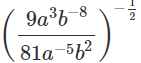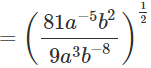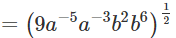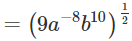= 3a−4b5

## Mathematics (Maths) Class 7

168 videos|252 docs|45 tests
 Use Code STAYHOME200 and get INR 200 additional OFF Use Coupon Code
Information about Important Questions: Exponents and Powers Page
In this test you can find the Exam questions for Important Questions: Exponents and Powers solved & explained in the simplest way possible. Besides giving Questions and answers for Important Questions: Exponents and Powers, EduRev gives you an ample number of Online tests for practice

## Mathematics (Maths) Class 7

168 videos|252 docs|45 tests